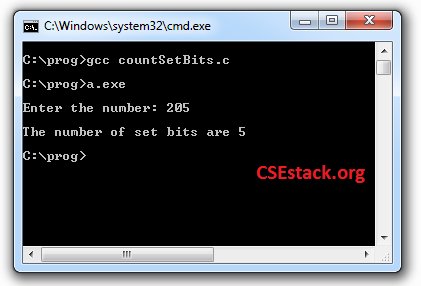# C Program to Count Number of Ones in Binary [Set bits in an Integer]

Problem Statement: You have given an integer number. You have to write a C Program to Count Number of Ones in Binary representation of a given integer number.

Note: One’s bit is also called as the set bit.

```Suppose you have integer value 13.
Its binary representation is 1101.
So the number of set bits in 13 is 3.```

Takes another example.

```Suppose the number is 205.
Its equivalent binary number is 11001101.
The total number of set bits in 205 is 5.```

Note: To convert the number from decimal to integer you can check out the online tool for Decimal to Binary.

Once you get the Binary equivalent to the decimal number, your task is…

#### How to count a number of ones in binary?

This question has been asked in the Riverbed interview and in many other interviews. It is somewhat tricky.

We know all, the computer (or any machine) only knows binary data i.e one or zero, on or off. The data we work on the computer is actually stored and retrieved in the binary form. So it is necessary many times to manipulate binary data. One of the clever examples is counting set bits in an integer value.

I kindly request you to minimize this window and try to get your logic for this question. If you don’t get any solution you can check the code below.

#### C Program to Count Number of Ones in Binary:

```#include<stdio.h>
void main()
{
int i=0;
int nNum;
int nCount=0;
printf("\nEnter the number: ");
scanf("%d",&nNum);
for(;i<(sizeof(int)*8);i++) { if(1&nNum) nCount++; nNum = nNum>>1;
}
printf("\nThe number of set bits are %d \n", nCount);
}

```

Here we are using right shift << bit operator.

#### How does the Right shift “<<” operator work?

It shifts the one-bit of the number to its right and adds zero bit at the left side.

The number 11001101 will become 01100110 after the one-bit right operation.

#### The output of the program:

```Enter the number: 205
The number of set bits are 5.```If you are new to the C programming you can check out the commands for compiling and executing C code.

Let’s take another challenge…

If you want to explore and learn more about, bit operations, here is another challenge for you.

This is the easiest method to calculate 2’s complement of any number. You should know this method if you want to do binary calculations very quickly.

If you have any questions understanding bit operation or regarding this challenge to count number of ones in binary number, write in a comment below.

Happy Programming!